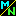Navigation Panel:(These buttons explained below)## What relevance do imaginary numbers have to the real world?

Okay, now we've seen that imaginary numbers exist. However, they exist in the context of a different number system, something different from the number systems we are used to. The "complex numbers" that make up this system are pairs of numbers; do they really deserve to be called "numbers" in their own right?

Well, remember that fractions are pairs of numbers also. They clearly deserve to be called numbers in their own right, since they can measure "how much" in some contexts (for instance, "I ate three quarters of a pie"). So, the principle of considering a pair of numbers (in this example, 3 and 4) as a number in its own right is well established.

The fact remains, though, that complex numbers have much less direct relevance to real-world quantities than other numbers do. An imaginary number could not be used as a measurement of how much water is in a bottle, or how far a kite has travelled, or how many fingers one has.

Nonetheless, there are a few real world quantities for which complex numbers are the natural model. The strength of an electromagnetic field is one example. The field has both an electric and a magnetic component, so it takes a pair of real numbers (one for the intensity of the electric field, one for the intensity of the magnetic field) to describe the field strength. This pair of real numbers can be thought of as a complex number, and it turns out that the strange rule of multiplication of complex numbers has relevance to the physics of an electromagnetic field.

Although such direct applications of complex numbers to the real world are few, their indirect applications are many. Many properties related to real numbers only become clear when the real numbers are thought of as sitting inside the complex number system. Therefore, complex numbers aid in the understanding even of things that are described by ordinary, familiar real numbers.

It's like trying to understand a shadow. The shadow lives in a two-dimensional world, so only two-dimensional concepts are directly applicable to it. However, thinking of the three-dimensional object casting the shadow can aid in understanding it, even though three-dimensional concepts don't have any direct application to the two-dimensional world of the shadow. Likewise, complex numbers may not be directly applicable to a real world measurement any more than a three-dimensional object is directly applicable to a 2-dimensional shadow, but they can still help us understand it.

Here's another analogy that may be helpful. Consider the following argument:

Population A has 236 people, 48 of them children.

Population B has 1234 people, 123 of them children.

The fraction of children in population A is 48/236 while the fraction of children in population B is 123/1234.

48/236 (approx. 0.2) is much greater than 123/1234 (approx. 0.1).

Therefore, population A is, on the whole, a much younger population than population B.

This argument has used fractions, non-integer numbers, in a problem where they have no physical relevance! You can't measure populations in fractions; you can't have "half a person", for example. The kind of numbers that have direct relevance to measuring numbers of people are the natural numbers; fractions are just as alien to this context as the complex numbers are alien to most real-world measurements. And yet, despite this, allowing ourselves to move from the natural numbers to the larger set of rational numbers enabled us to deduce something about the real world situation, even though measurements in that particular real world situation only involve natural numbers.

In the same way, being willing to think about what happens in the larger set of complex numbers allows us to draw conclusions about real world situations even when actual measurements in that particular real world situation only involve the real numbers. This happens all the time in engineering applications. If you've seem some calculus, you may be able to understand the following example: in engineering and other applications, one very often has to solve an equation of the form a y" + b y' + c y = 0 (*) for the unknown function y.

It turns out that there's a way to get the solutions provided one can solve the quadratic equation a r^2 + b r + c = 0 for the variable r. In the real numbers, there may not be any solutions. However, in the complex numbers there are, so one can find all complex-valued solutions to the equation (*), and then finally restrict oneself to those that are purely real-valued. The starting and ending points of the argument involve only real numbers, but one can't get from the start to the end without going through the complex numbers. Since equations like (*) need to be solved all the time in real-life applications such as engineering, complex numbers are needed.

Navigation Panel: